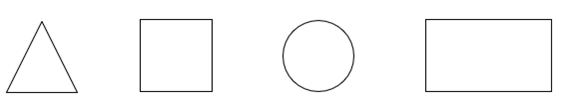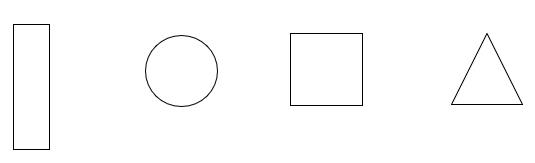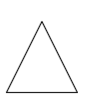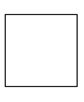Unit 4: Estimating, Time, and Shapes

# Unit 4 Review: Estimating, Time, and Shapes

You will now practice all the skills you learned in Unit 4. Check your work using the answer key at the end of the review.

1. Estimate the following answers. Be sure to round to the nearest 10 before adding.
1. \begin{align}23\\+32\\ \hline \\ \end{align}
2. \begin{align}68\\+17\\ \hline \\ \end{align}
3. \begin{align}34\\+28\\ \hline \\ \end{align}
4. \begin{align}42\\+53\\ \hline \\ \end{align}
5. \begin{align}74\\+24\\ \hline \\ \end{align}
6. \begin{align}33\\+28\\ \hline \\ \end{align}
7. \begin{align}17\\+42\\ \hline \\ \end{align}
8. \begin{align}27\\+18\\ \hline \\ \end{align}
2. Estimate the following sums. Be sure to round to the nearest 10 before adding.
1. \begin{align}625\\+254\\ \hline \\ \end{align}
2. \begin{align}432\\+325\\ \hline \\ \end{align}
3. \begin{align}328\\+163\\ \hline \\ \end{align}
4. \begin{align}529\\+248\\ \hline \\ \end{align}
5. \begin{align}536\\+137\\ \hline \\ \end{align}
6. \begin{align}867\\+215\\ \hline \\ \end{align}
7. \begin{align}843\\+107\\ \hline \\ \end{align}
8. \begin{align}435\\+127\\ \hline \\ \end{align}
3. Estimate the following answers. Be sure to round to the nearest 10 before subtracting.
1. \begin{align}43\\-28\\ \hline \\ \end{align}
2. \begin{align}64\\-25\\ \hline \\ \end{align}
3. \begin{align}73\\-47\\ \hline \\ \end{align}
4. \begin{align}83\\-24\\ \hline \\ \end{align}
5. \begin{align}68\\-28\\ \hline \\ \end{align}
6. \begin{align}54\\-22\\ \hline \\ \end{align}
7. \begin{align}67\\-29\\ \hline \\ \end{align}
8. \begin{align}85\\-29\\ \hline \\ \end{align}
4. Estimate the following answers. Be sure to round to the nearest 10 before subtracting.
1. \begin{align}625\\-407\\ \hline \\ \end{align}
2. \begin{align}908\\-413\\ \hline \\ \end{align}
3. \begin{align}976\\-134\\ \hline \\ \end{align}
4. \begin{align}882\\-257\\ \hline \\ \end{align}
5. \begin{align}572\\-154\\ \hline \\ \end{align}
6. \begin{align}908\\-713\\ \hline \\ \end{align}
7. \begin{align}965\\-702\\ \hline \\ \end{align}
8. \begin{align}988\\-254\\ \hline \\ \end{align}
5. Write the following times in standard format.
1. 10 hours, 20 minutes, 12 seconds
2. 8 hours,45 minutes, 6 seconds
3. 5 hours, 32 minutes, 45 seconds
4. 1 hour, 7 minutes, 28 seconds
5. 12 hours, 55 minutes
6. 6 hours, 5 minutes, 39 seconds
6. Write the following times using a.m. or p.m.
1. The movie starts at 6:45 in the evening.
2. Your friend calls and wakes you up at 3:23 in the morning.
3. Your dog barks at the mailman at 2:35 in the afternoon.
4. Your morning break is at 10:15.
7. Round the following times to the nearest hour.
1. You took a walk for 47 minutes.
2. Your round trip (there and back) to the mall took 2 hours and 12 minutes.
8. Circle the correct shape in each line.
1. A triangle2. A square9. The following things give the idea of a shape. Write the name of the shape in each blank.
1. A window is a          .
2. A checkerboard is a          .
3. A watch is a          .
4. A yield sign is a          .
10. Word Problems. Estimate the following answers. Be sure to round to the nearest 10 before adding or subtracting. Be sure to circle the information and underline what’s being asked.
1. The Sears Tower is 443 metres tall. It has a 105 metre TV antenna on top. Estimate the height of the building and the antenna.
2. A restaurant used 76 kilograms of potatoes and 68 kilograms of meat. Estimate how many kilograms of potatoes and meat the restaurant used altogether.
3. Paolo’s father weighs 78 kilograms. Paolo weighs 29 kilograms. Estimate how much more Paolo’s father weighs.
4. Chi bought 54 litres of gasoline on Tuesday. He ought 38 litres of gasoline on Friday. Estimate how many litres of gas he bought altogether.

# Answers to Unit 4 Review

1. 50
2. 90
3. 60
4. 90
5. 90
6. 60
7. 60
8. 50
1. 880
2. 760
3. 490
4. 780
5. 680
6. 1090
7. 950
8. 570
1. 10
2. 30
3. 20
4. 60
5. 40
6. 30
7. 40
8. 60
1. 220
2. 500
3. 850
4. 620
5. 420
6. 200
7. 270
8. 740
1. 10:20:12
2. 8:45:06
3. 5:32:45
4. 1:07:28
5. 12:55
6. 6:05:39
1. 6:45 p.m.
2. 3:23 a.m.
3. 2:35 p.m.
4. 10:15 a.m.
1. 1 hour
2. 2 hours
1.2.1. rectangle
2. square
3. circle
4. triangle
1. 550 metres
2. 50 kilograms
3. 150 kilograms
4. 90 litres